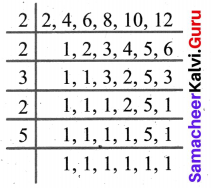## Tamilnadu Samacheer Kalvi 6th Maths Solutions Term 2 Chapter 1 Numbers Additional Questions

Question 1.
A pair of prime numbers whose difference is 2, is called _____
Solution:
Twin primes

Question 2.
The first 4 digit number divisible by 3 ______
Solution:
1002

Question 3.
Prime triplet ______
Solution:
(3, 5, 7)

Question 4.
100 years = ______
Solution:
100 years = 1 Century

Question 5.
Loss = ____
Solution:
Loss = CP – SP

Question 6.
526 ml _____
Solution:
526 ml = 0.526 L

Question 7.
No sides are equal _____
Solution:
Scalene TriangleQuestion 8.
What is the total number of primes up to 100?
Solution:
25

Question 9.
Check whether (37, 39) is a twin prime?
Solution:
No, because 39 is not a prime number.

Question 10.
Check the divisibility by 11 of 684398?
Solution:
In 684398
Sum of digits in odd places = 8 + 3 + 8 = 19
Sum of digits in even places = 6 + 4 + 9 = 19.
Difference = 19 – 19 = 0.
684398 is divisible by 11.

Question 11.
Is 53249624 is divisible by 8? How?
Solution:
In 53249624, consider the last three digits 624, which is divisible by 8.
53249624 is divisible by 8.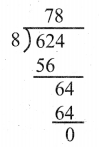Question 12.
Factorise 1056
Solution: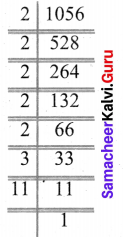1056 = 2 × 2 × 2 × 2 × 2 × 3 × 11

Question 13.
Express 42 and 100 as the sum of two consecutive primes?
Solution:
42 = 19 + 23
100 = 47 + 53Question 14.
A heap of stones can be made up into groups of 21. When made up into groups of 16, 20, 25 and 45 there are 3 stones left in each case. How many stones at least can there be in the heap?
Solution:
LCM of 16, 20, 25, 45 = 2 × 5 × 2 × 5 × 2 × 2 × 3 × 3 = 3600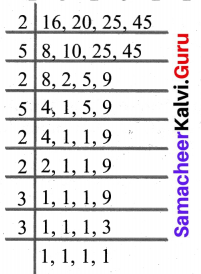The heap contain 3600 + 3 = 3603 stones at least.
3603 stones at least can there be in the heap.

Question 15.
Find the largest number of four digits exactly divisible by 12, 15, 18 and 27
Solution:
The largest number of four digits = 9999
lcm of 12, 15, 18, 27 is 540
Dividing 9999 by 540
We get 279 as the remainder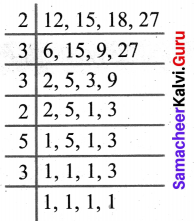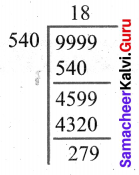LCM = 2 × 3 × 3 × 2 × 5 × 3 = 540
Required number = 9999 – 279 = 9720

Question 16.
Find the least number which when divided by 6, 7, 8, 9 and 12 leaves the same remainder 1 in each case.
Solution:
Required number = [LCM (6, 7, 8, 9, 12)] + 1
LCM (6, 7, 8, 9, 12) = 3 × 2 × 2 × 2 × 3 × 7 = 504
Required number = 504 + 1 = 505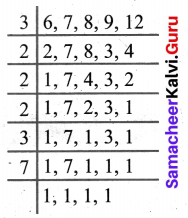Question 17.
Product of two co-prime numbers is 117. Then what will be their LCM?
Solution:
We know that LCM × HCF = Product of two numbers
Also, we know that HCF of two co-primes = 1
LCM × 1 = 117
LCM = 117

Question 18.
Six bells commence tolling together and toll at intervals of 2, 4, 6, 8, 10 and 12 seconds respectively. In 30 minutes, how many times do they toll together?
Solution:
LCM of 2, 4, 6, 8, 10 and 12 is 120
So the bell will toll together after every 120 seconds i.e 2 minutes.
In 30 minutes, they will toll together $$\frac{30}{2}$$ + 1 = 16 times.
LCM = 2 × 2 × 3 × 2 × 5 = 120